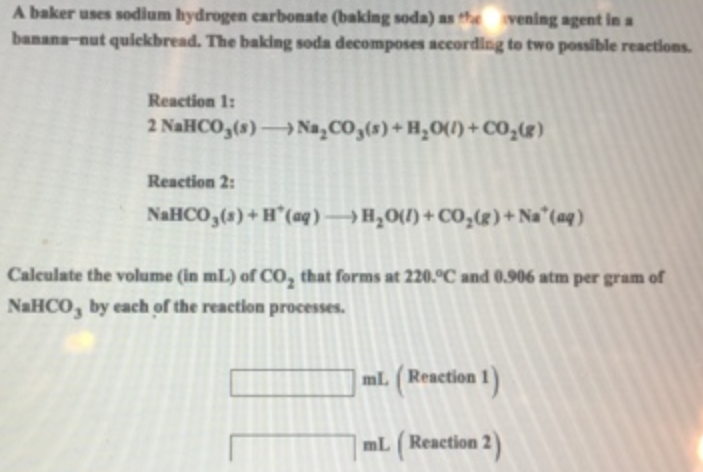### Learn how to solve problems like this one with our expert tutor.

🤓 Based on our data, we think this question is relevant for Professor Dunn's class at KU.

# Solution: A baker uses sodium hydrogen carbonate (baking soda) as the agent in a banana-nut quickbread. The baking soda decomposes according to two possible reactions. Reaction 1: 2NaHCO3(s) → Na2CO3(s) + H2O(l) + CO2(g) Reaction 2: NaHCO3(s) + H+(aq) → H2O(l) + CO2(g) + Na+(aq) Calculate the volume (in mL) of CO2 that forms at 220 °C and 0.906 atm per gram of NaHCO 3 by each of the reaction processes.

###### Problem

A baker uses sodium hydrogen carbonate (baking soda) as the agent in a banana-nut quickbread. The baking soda decomposes according to two possible reactions.

Reaction 1: 2NaHCO3(s) → Na2CO3(s) + H2O(l) + CO2(g)

Reaction 2: NaHCO3(s) + H+(aq) → H2O(l) + CO2(g) + Na+(aq)

Calculate the volume (in mL) of CO2 that forms at 220 °C and 0.906 atm per gram of NaHCO 3 by each of the reaction processes.View Complete Written Solution